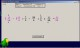KevPT 2.5.1 KevPT can be used to track problems, questions, or requests from you or your clients. SharewareMaster Math Word Problems 1.2 Master Math Word Problems is an easy to use program to aide students in learning to identify key words that identify mathematical operations and work through mathematical word problems. SharewareMaster Math Word Problems X 1.8.1 Master Math Word Problems is an easy to use program to aid students in learning to identify key words that identify mathematical operations and work through mathematical word problems. SharewareDoktor Kleanor 10.4 Doktor Kleanor helps you to easily solve common problems experienced while running Mac OS X 10. FreewareCircles 1.0 The program contains thirty-two pre-made circle area problems, and thirty-two pre-made circumference problems. FreewareInternetMath 7 Uses the Internet to teach how to solve math word problems grades 1-8 SharewareInternet Math 1.0 Uses the Internet to teach how to solve math word problems grades 1-8 SharewareNero TuneItUp Free 2.8.0.84.0 Nero TuneItUp finds and fixes the PC problems that slow you down. FreewareAbilities Builder Add & Subtract Fractions 3.1 Build fraction addition and subtraction skills for simple to complex problems. DemoAbilities Builder Fractions Plus 1.0 Add, subtract, multiply and divide simple to complex fraction problems. Demo